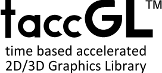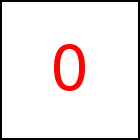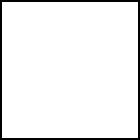# acceleration Library Method

Short Description: Specify Acceleration as Vector

Signature: t.acceleration (a) or (x,y,z) or (x,y)
Group: Motion
Class: transition Class

Explicitly sets the acceleration used for a motion with constant acceleration. Please refer to Motions for an explanation of motion with constant acceleration. Default acceleration is zero. This means that the objects moves with constant speed throughout the transition.

Sample HTML element with id="sample"

Acceleration can be given as a scalar value a or as a 2D vector x,y or 3D vector x,y,z. In case of a scalar the acceleration works towards (or against if a< 0) the direction of the motion. I.e. if the acceleration is positive velocity increases throughout the transition and decreases for negative acceleration.

 taccgl.actor("sample") . to({oy:500}). dur(5). acceleration(30) . start() RUN taccgl.actor("sample") . to({oy:500}). dur(5). acceleration(-30). start() RUN taccgl.actor("sample") . to({oy:500}). dur(5). acceleration(40). start() RUN taccgl.actor("sample") . to({ox:-350}). dur(5). acceleration(30) . start() RUN taccgl.actor("sample") . to({ox:-350}). dur(5). acceleration(-30). start() RUN

taccgl™ determines the begin and end velocity automatically based on the start and end point, on the duration and the acceleration. So typically if the acceleration is increased taccgl™ automatically reduces the starting velocity to reach the end point within the specified duration.

Note that either starting or end point or both have to be set (e.g. using from or to). Also the duration needs to be set before. Also note that the acceleration may be set just once using one of the functions acceleration, vBegin, and vEnd.

If the accelecation is increase beyond a certain point, staring velocity might even get negative:

 taccgl.actor("sample") . to({oy:500}). dur(5). acceleration(50). start() RUN taccgl.actor("sample") . to({oy:500}). dur(5). acceleration(80). start() RUN

Acceleration can be given in vector form. If a 2D vector is given it is extended to 3D by adding z=0. The examples below show various values and directions for the initial velocity and the acceleration. If acceleration is given in vector form start and end point of a motion can be identical (2nd example below).

 taccgl.actor("ball") . to({ox:-500}). dur(2). acceleration (0,500,0). start() RUN taccgl.actor("ball") . dur(2). acceleration (0,500,0). start() RUN taccgl.actor("ball") . to({ox:-500}). dur(2). acceleration (0,0,-4000). start() RUN taccgl.actor("ball") . to({ox:-500}). dur(2). acceleration (1500,3000,8000). start() RUN
Next Page:transition.vBegin - Set initial velocity
Previous Page: transition.flyToElement - Move object to HTML Element

# Motion

taccgl™ - Canvas Animation Library